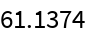#Function Repository Resource:

# BitListToByteArray

Convert a list of bits to a byte array

Contributed by: Arnoud Buzing
 ResourceFunction["BitListToByteArray"][bits] converts the list bits to a ByteArray.

## Details and Options

The output typically requires 64 times less memory than the input.

## Examples

### Basic Examples

Create a list of bits:

 In:=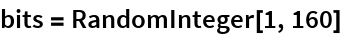Out=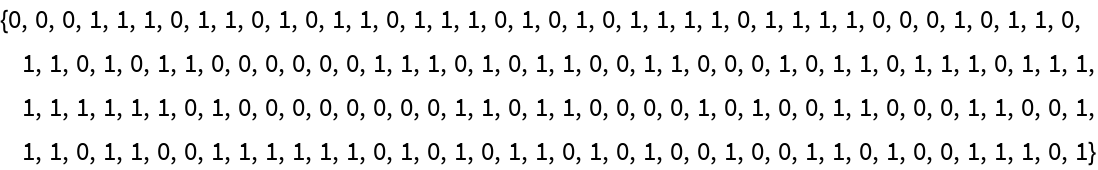Convert to a byte array:

 In:=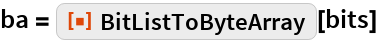Out=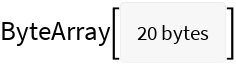Compare the number of bytes needed to represent the expressions:

 In:=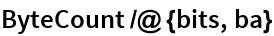Out=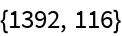For larger bit arrays, the relative memory compression does approach a factor of 64:

 In:=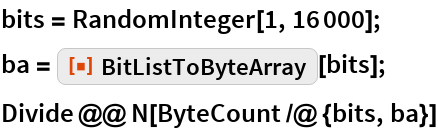Out=